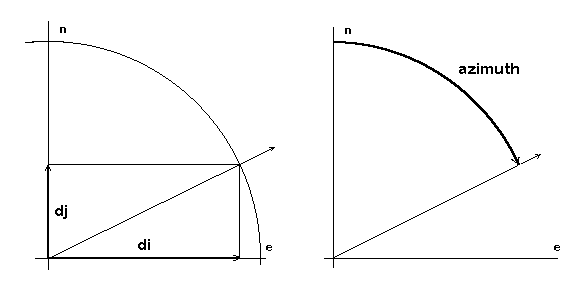Retrun to document top page

### Nemo Library, section 2

Simple Algebra and Geometry
```   Simple utilities, performing elementary geomerty computations, used  by
the functions in the "higher level" library sections.
```

 nemo_NormalizeV2() Normalize generic two-componenet vector nemo_NormalizeV3() Normalize generic three-componenet vector nemo_DirectionToAzimuth() Azimuth (angle) from direction cosines nemo_MidV3() Find mid-vector in 3D space nemo_ArcV3() Spherical arc nemo_ChordSqToArcApprox() Square chord to approximate arc nemo_ArcToChordApprox() Arc to approximate chord nemo_LatLongToDcos3() Latitude/longitude to generic vector nemo_Dcos3ToLatLong() Generic 3D vector to latitude/longitude

nemo_NormalizeV2()
```Synopsis:
#include <nemo.h>
void nemo_NormalizeV2(double v);
Description:
Normalize a vector in 2 dimensional space.
Argument:
v: An array of two doubles, values given and updated, the vector to be
normalized (i.e., its magnitude made equal to 1.0). If the magnitude
of the given vector is near-zero, both components will be set to
NEMO_DOUBLE_UNDEF value.
nemo_NormalizeV3()```

nemo_NormalizeV3()
```Synopsis:
#include <nemo.h>
void nemo_NormalizeV3(double v);
Description:
Normalize a vector in 3 dimensional space.
Argument:
v: An array of three doubles, values given and updated, the vector to be
normalized (i.e., its magnitude ne made equal to 1.0). If the magnitude
of the given vector is near-zero, all three components will be set to
NEMO_DOUBLE_UNDEF value.
nemo_NormalizeV2()```

nemo_DirectionToAzimuth()
```Synopsis:
#include <nemo.h>
double nemo_DirectionToAzimuth(const double dx);
```Planar direction represented by its two vector componnets (di, dj) and by the azimuth angle

```Description:
Given a planar direction as two direction cosines, return the azimuth as
an angle, measured in radianas, from Y/North ditection in mathematically
negative rotation sense (i.e., "cloclwise from North" - this is the common
definition of "azimuth" in surveying, mapping, navigation etc).
If α is the azimuth angle so defined, direction cosines commonly used
to manipulate planar directions in the Library (di, dj) are:
di = cos(π/2 - α) and dj = sin(π/2 - α).
Argument:
pDx:
Pointer to an array of two doubles, ordered pair of di and dj direction
cosines.
Return Value:
double, angle in radians, azimuth measured clockwise from North.
```

nemo_MidV3()
```Synopsis:
#include <nemo.h>
void nemo_MidV3(const double va, const double vb, double vm);
Description:
Find a 3D vector that is a mid-vector between two given ones.
Arguments:
va:
An array of three doubles, first given vector.
vb:
An array of three doubles, second given vector.
vmid:
An array of three doubles, returned (normalized) mid-vector.
nemo_NormalizeV2()   nemo_NormalizeV3()```

nemo_ArcV3()
```Synopsis:
#include <nemo.h>
double nemo_ArcV3(const double va, const double vb);
Description:
Compute the length of spherical arc between two unit sphere vectors.
Arguments:
va:
An array of three doubles, first given vector.
vb:
An array of three doubles, second given vector.
Return Value:
Double, length of arc between va and vb.
nemo_MidV3()```

nemo_ChordSqToArcApprox()
```Synopsis:
#include <nemo.h>
double nemo_ChordSqToArcApprox(double chordSq);
Description:
Compute the length of circular arc, given the squared length of its chord.
The computation is based on the arcsine series expansion; it is therefore
approximate but of sufficient precision for use by all higher-level Nemo
Library functions.
Argument:
chordSq:
Given squared legth of a chord on a unit circle for which the arc
length is required.
Return Value:
Double, length of the arc on the unit circle.
nemo_ArcToChordApprox()   nemo_ArcV3()```

nemo_ArcToChordApprox()
```Synopsis:
#include <nemo.h>
double nemo_ArcToChordApprox(double arc);
Description:
Given the length of an arc on a unit circle, compute the length of
it's chord. The computattion is based on the sine series expansion;
it is therefore approximate, but of sufficient precision for use by
all higher-level Nemo Library functions.
Argument:
arc:
Double, length of an arc on a unit circle for which the chord length
is required.
Return Value:
Double, length of the chord on the unit circle.
nemo_ChordSqToArcApprox()   nemo_ArcV3()```

nemo_LatLongToDcos3()
```Synopsis:
#include <nemo.h>
void nemo_LatLongToDcos3(const double ang, double vect);
Description:
Convert generic latitude/longutude to a direction cosines.
Arguments:
ang:
An array of two doubles, given latitude/longitude.
vector:
An array of three doubles, returned direction cosines.
nemo_Dcos3ToLatLong()```

nemo_Dcos3ToLatLong()
```Synopsis:
#include <nemo.h>
void nemo_Dcos3ToLatLong(const double vect, double ang);
Description:
Convert generic direction cosines to latitude/longutude.
Arguments:
vector:
An array of three doubles, given direction cosines.
ang:
An array of two doubles, returned latitude/longitude.